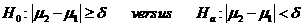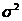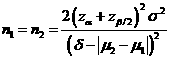# Sample Size Calculator: Two Parallel-Sample Means

Hypothesis: One-Sided EquivalenceData Input: (Help) (Example)

 Input Results α β Allowable difference n Population variance δ

Note:

 Variables Descriptions α One-sided significance level 1-β Power of the test Allowable difference Acceptable mean difference between sample two and sample one (µ2-µ1) Population variance Population varianceδ Equivalence limit n Sample size of each group

Help Aids Top

Application: This procedure is used to test the following hypotheses:The test drug is concluded to be equivalent to the control in average if the null hypothesis is rejected at significance level α.

Procedure:

1. Enter

a)      value of α, the probability of type I error

b)      value of β, the probability of type II error

c)      value of allowable difference

d)     value of Population variance

e)      value of δ>0, the equivalence limit.

1. Click the button “Calculate” to obtain result sample size of each group n.

Formula:(*)

Notations:

α:           The probability of type I error is the Probability of rejecting the null hypothesis when null hypothesis is true. The null hypothesis is the two mean values are not equivalent.

β:           The probability of type II error is the Probability of failing to reject the null hypothesis when null hypothesis is false.

δ:           The largest change from the reference value (baseline) that is considered to be trivial.

μ2 – μ1:  Value of allowable difference is the true mean difference between a test drug (μ2) and a placebo control or active control agent (μ1).

Examples

Example 1: Suppose the true difference is 1% (i.e., μ2–μ1=1%) and the equivalence limit is 5% (i.e., δ=0.05). Thus, by using (*), with the standard deviation is 10% (i.e., population variance is 0.01), the required sample size to achieve an 80% power (β=0.2) at α=0.05 for correctly detecting such difference of 0.05 change obtained by normal approximation as n=108.

Reference: Chow, Shao and Wang, Sample Size Calculations In Clinical Research, Taylor & Francis, NY. (2003) Pages 59-61.

Top